It is given a quadratic function y = -4x2+5x+c with unknown coefficient c. Determine the smallest integer c for which the graph of f intersects the x-axis at two different points.

c =  0

### Step-by-step explanation:

Our quadratic equation calculator calculates it.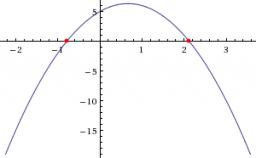Did you find an error or inaccuracy? Feel free to write us. Thank you!Tips to related online calculators

## Related math problems and questions:

• A Cartesian framework1. In a Cartesian framework, the functions f and g we know that: the function (f) is defined by f (x) = 2x ^ 2, the function (g) is defined by g (x) = x + 3, the point (O) is the origin of the reference, point (C) is the point of intersection of the graph
• LineStraight-line passing through points A [-3; 22] and B [33; -2]. Determine the total number of points of the line in which both coordinates are positive integers.
• Function 3Function f(x)=a(x-r)(x-s) the graph of the function has x- intercept at (-4, 0) and (2, 0) and passes through the point (-2,-8). Find constant a, r, s.
• Coordinate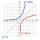Determine missing coordinate of the point M [x, 120] of the graph of the function f bv rule: y = 5xWrite the equation of the quadratic function, which includes points A (-1, 10), B (2, 19), C (1,4)Which of the points belong function f:y= 2x2- 3x + 1 : A(-2, 15) B (3,10) C (1,4)
• 600 pencils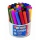600 pencils we want to be divided into three groups. The biggest groups have ten pens more than the smallest. How many ways can this be done?
• RectangleThe perimeter of the rectangle is 22 cm and content area 30 cm2. Determine its dimensions, if the length of the sides of the rectangle in centimeters is expressed by integers.
• Roots and coefficient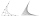In the equation 2x ^ 2 + bx-9 = 0 is one root x1 = -3/2. Determine the second root and the coefficient b.
• Points on circleIn the Cartesian coordinate system with the origin O is a sketched circle k /O; r=2 cm/. Write all the points that lie on a circle k and whose coordinates are integers. Write all the points that lie on the circle I / O; r=5 cm / and whose coordinates are
• Sinus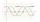Determine the smallest integer p for which the equation 4 sin x = p has no solution.
• Exponential equationDetermine the value of having y in the expression (3^y): (4^-1)=36. Unknown y is a natural number greater than zero.
• Diofant equationIn the set of integers (Z) solve the equation: 212x +316y =0 Write result with integer parameter t in Z (parameter t = ...-2,-1,0,1,2,3... if equation has infinitely many solutions)
• Four integersFnd four consecutive integers so that the product of the first two is 70 times smaller than the product of the next two.
• IntegerFind the integer whose distance on the numerical axis from number 1 is two times smaller as the distance from number 6.
• Five numbers in ratioFive integers are in the ratio 1: 2: 3: 4: 5. Their arithmetic mean is 12. Determine the smallest of these numbers.
• Unknown number 7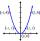Calculate unknown number whose 12th power when divided by the 9th power get a number 27 times greater than the unknown number. Determine the unknown number.